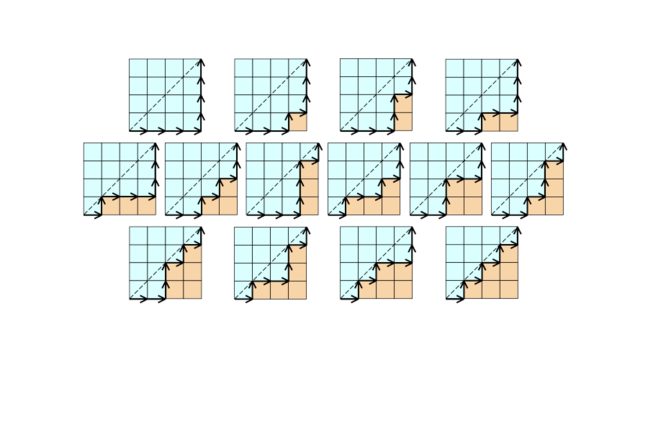## Want to keep learning?

This content is taken from the Davidson Institute of Science Education at the Weizmann Institute of Science's online course, Flexagons and the Math Behind Twisted Paper. Join the course to learn more.
3.8

## Davidson Institute of Science Education at the Weizmann Institute of ScienceThe North and East grid paths between 2 points that do not cross the diagonal between them

# Flexagons and Catalan numbers

Catalan numbers are one of my favourite types of numbers! They pop up regularly in situations where things need to be counted (a branch of mathematics called combinatorics).

### Catalan numbers

Here is a list of the first ten Catalan numbers:

• 1, 1, 2, 5, 14, 42, 132, 429, 1430, 4862…

Take for example the following problem. You live in a city built like a grid, like Manhattan or Toronto. To get to work you need to walk four blocks East and four blocks North. How many different ways are there to do this, if you are not allowed to cross the diagonal line between your house and your work?

Pause. I’m waiting for you to figure this out…

Correct! The answer is 14. You can see all the different 14 paths in the image above. 14 is the fourth Catalan number. Actually, it’s the fifth, but we call the first number the zero’th, so that works out OK. If the distance between your house and work was five blocks South and five blocks West, the answer would be 42 different paths. If the distance was two blocks North and two blocks West, the answer would be 2. Generally speaking, for a grid with dimensions n x n, the answer is the n’th Catalan number. If you lived at work, the grid has the dimensions 0 x 0 and the answer would be the 0’th Catalan number which is one, so that makes sense.

Catalan numbers are also the answer to many other question such as: How many ways are there to divide a convex polygon with n + 2 sides into non-crossing triangles? For a hexagon, n = 4 and the answer is the 4’th Catalan number, 14.For the mathematically inclined there is a formula to calculate the Catalan numbers and you can find it here or here along with more examples and explanations.

### Catalan numbers and flexagons

So what does all this have to do with flexagons? For one, Catalan numbers pop up in combinatorial formulas for calculating the number of different kinds of flexagons. Here are 10 various number series, all related to some counting of flexagons, and all with some relationship to Catalan numbers. The most immediate connection though, is this.

A hexaflexagon pat can be split with a finger right through it so it comes out the other side. This splits the pat into two sub-pats. If there are only 2 leaves, as is the case with the tri-hexa-flexagon, there is only one way to split them. We’ll we’ll call the two leaves a and b, so we can write the pat structure as: (a,b), where the comma marks the splitting point. If there are 3 leaves in a pat, a, b and c, it can be split in two different ways: one leaf in the top sub-pat and two in the bottom, or two leaves in the top sub-pat and one in the bottom: (a,b-c) or (a-b,c). The dashed line means that the leaves are hinged. They create a pocket but not a full opening. Notice that because of the way flexagons are constructed, there can only be 1 or 2-leaf pats. This means that, when writing all the possible structures of a pat, we use all possible reductions to one or two leaf sub-pats. Hence, a four-leaf pat can be split in 5 different ways: (a, (b-c)-d); (a, b-(c,d)); (a-b,c-d); (a-(b-c),d); ((a-b)-c,d). Note that the difference between (b-c)-d and b-(c-d) is equivalent to the two options of the three leaf pat, except that instead of having a full opening there is an inner pocket. So (b-c)-d indicates a hinge between a two-leaf sub-pat on the top hinged to a single leaf on the bottom, and b-(c-d) indicates a hinge between a leaf on the top hinged to a two-leaf sub-pat on the bottom. A five-leaf pat can be split this way in 14 different ways. 1,2,5,14… Wait a minute! These are Catalan numbers! That’s the connection! In general, an (n+1) leaf pat can be split in the n’th Catalan number different ways…